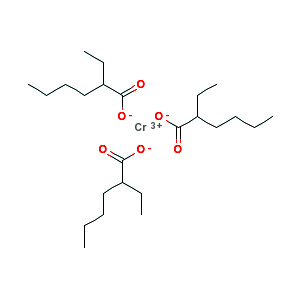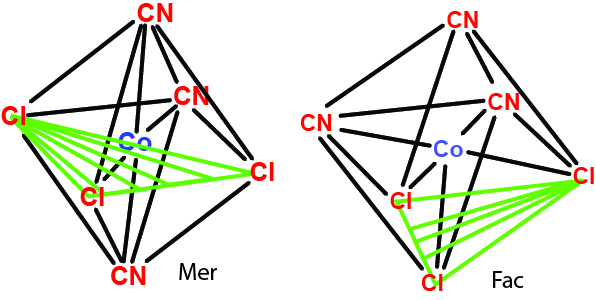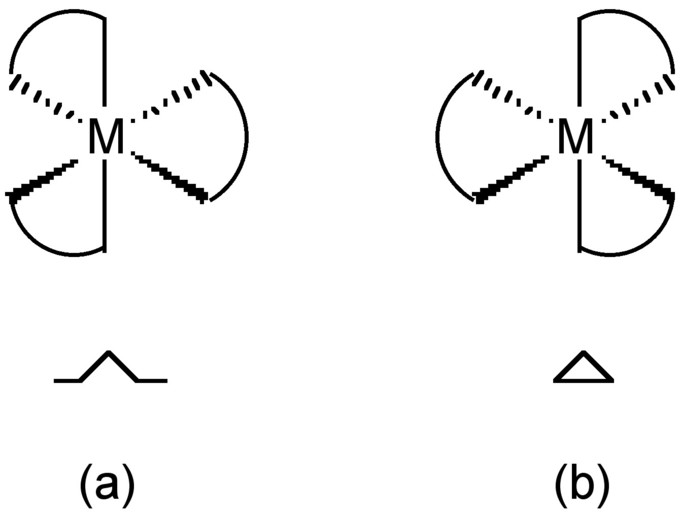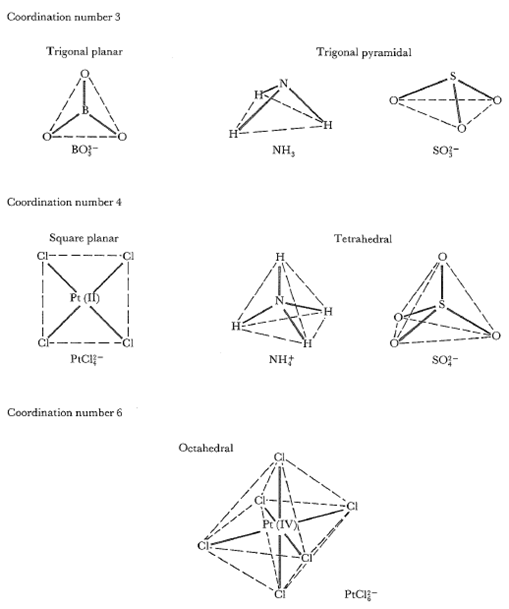## Oxidation Numbers of Metals in Coordination Compounds

Transition metals typically form several oxidation states and therefore have several oxidation numbers.

### Learning Objectives

Calculate the oxidation state of a metal in a coordination compound.

### Key Takeaways

#### Key Points

• The oxidation number of an atom is the charge that would exist on the atom if the bonding were completely ionic.
• In simple ions, the oxidation number of the atom is the charge on the ion.
• In a molecule or compound, the oxidation number is the sum of the oxidation numbers of its constituent atoms.
• Atoms with d-shell electrons can have several different oxidation numbers.
• In complex ions or molecules, the oxidation numbers of these atoms can be calculated if we assume that the oxidation numbers of the other atoms in the species are fixed.
• The oxidation numbers of metals with more than one oxidation state are represented by Roman numerals.

#### Key Terms

• oxidation number: The net sum of the negative, less the positive, charges on an atom.
• roman numeral: A numeral represented using a Roman system involving the letters I, V, X, L, C, D, and M.
• 18 electron rule: Rule used primarily for predicting formula for stable metal complexes; transition metals can accomodate at most 18 electrons in their valence shells.

### Oxidation Numbers

The oxidation number, or oxidation state, of an atom is the charge that would exist on the atom if the bonding were completely ionic. This oxidation number is an indicator of the degree of oxidation (loss of electrons) of an atom in a chemical compound. Conceptually, the oxidation state, which may be positive, negative or zero, is the hypothetical charge that an atom would have if all bonds to atoms of different elements were 100% ionic, with no covalent component. Oxidation number are typically represented by small integers.

In simple ions, the oxidation number of the atom is the charge on the ion. For example, Na+, K+, and H+ all have oxidation numbers of +1. O2- and S2- have oxidation numbers of -2. In a molecule or compound, the oxidation number is the sum of the oxidation numbers of its constituent atoms.

Example: What is the oxidation number of C2H6 ?

Solution: The oxidation number of C is -3. The oxidation number of H is +1 (H+ has an oxidation number of +1). For the compound, we calculate the oxidation number as follows: 2(-3) + 6(+1) = 0. Therefore, the oxidation number of C2H6 is 0.

Many atoms, including most atoms with d subshells, can have several different oxidation numbers. In complex ions or molecules, the oxidation numbers of these atoms can be calculated if we assume that the oxidation numbers of the other atoms in the species are fixed.Oxidation number of ethane: Oxidation numbers of carbon and hydrogen in ethane.

### Oxidation Numbers of Transition Metals

The oxidation number in coordination chemistry has a slightly different meaning. Transition metals are the elements in Groups 3 to 12 representing the d block of the periodic table. Due to the relatively low reactivity of unpaired d electrons, these metals typically form several oxidation states and therefore can have several oxidation numbers. Unfortunately, there is no simple rule to determining oxidation state possibilities among the transition metals, so it is best simply to memorize the common states of each element.

Example 1: An oxide of chromium has the formula Cr2O3. What is the oxidation number of each chromium ion?

Solution: The oxidation number of oxygen is assigned a charge of -2 when it reacts with a metal because the metal is more electropositive. The total charge of the oxygens is -6. Since the compound is neutral, the charge of each chromium must be +3 because 2(3)+3(-2) = 0.

Example 2: Another compound has the formula K2CrO4. What is the oxidation number of chromium?

Solution: The oxidation number for oxygen is assigned a charge of -2 when it reacts with a metal. Because there are 4 oxygen atoms, the total charge of the oxygens is -8. Potassium has an oxidation number of +1, giving an overall charge of +2. Because the compound is neutral and 2(1)+(Cr)+4(-2)=0, chromium must have an oxidation number of +6.

### Multiple Oxidation States

The oxidation number for metals that can have more than one oxidation state is represented by a Roman numeral. The oxidation number is placed in parentheses after the name of the element (iron(III)). Sometimes, the oxidation states can also be written as a superscripted number to the right of the element symbol (Fe3+).

The maximum oxidation number in the first row of transition metals is equal to the number of valence electrons from scandium (+3) up to manganese (+7). However, it decreases in the latter elements. In the second and third rows, the maximum oxidation number is that of ruthenium and osmium (+8). In compounds such as (MnO4) and OsO4, the elements achieve a stable octet by forming four covalent bonds.

### Oxidation Numbers of Main-Group Elements

Main-group elements, those in Groups 13 to 17, also exhibit multiple oxidation states. The “common” oxidation states of these elements typically differ by two. For example, in compounds containing gallium the oxidation states of gallium are +1 and +3. No compound of gallium(II) is known; any such compound would have an unpaired electron and would behave as a free radical and be destroyed rapidly. The only compounds in which gallium has a formal oxidation state of +2 are dimeric compounds, such as [Ga2Cl6]2−, which contain a Ga -Ga bond formed from the unpaired electron on each Ga atom.

## Naming Coordination Compounds

Transition-metal and coordination compounds are named using a set of rules that describe oxidation numbers and anion and cation composition.

### Learning Objectives

Identify the proper name for a coordination complex given its molecular formula.

### Key Takeaways

#### Key Points

• When you are naming a transition- metal compound, it is necessary to indicate which oxidation number the metal has.
• The oxidation number appears as a Roman numeral in parentheses after the cation.
• Coordination compounds contain an ion and an array of ligands.
• Naming coordination compounds involves a set of rules which includes the oxidation number of the central ion and the chemical composition of the ligand, focusing on the anionic and cationic properties of the molecule.

#### Key Terms

• polyatomic: Of a molecule or ion consisting of three or more atoms.
• polydentate: Coordinated to the central atom by several bonds; multidentate.
• monodentate: Describing a ligand that has only a single bond with the central atom.
• coordination compound: A class of compounds in which a central metal atom (normally a transition element) is surrounded by a group of ions or molecules (ligands).

### Naming Transition Metals

Many transition metals exist as more than one type of cation. For example, iron exists as Fe2+ and Fe3+. Therefore, when you are naming an ionic compound containing iron, it is necessary to indicate which oxidation number the metal has. The oxidation number appears as a Roman numeral in parenthesis after the cation. For metals, the oxidation number is the same as the charge. Fe2+ in a compound with chloride would have a formula FeCl2 and would be named iron(II) chloride. The cation Fe3+ paired with oxygen would have the formula Fe2O3 and would have the name iron(III) oxide.

The procedure for naming ionic compounds containing polyatomic ions is the same as that for naming simple ions. Thus, CaCO3 is named calcium carbonate; (NH4)2HPO4, a compound with two polyatomic ions, is named ammonium hydrogen phosphate; and Pb2+ paired with SO42- (PbSO4) is named lead(II) sulfate.

Example 1: Write a correct chemical formula for each of the following ionic compounds: a. Aluminum oxide; b. Copper (II) chloride; c. Iron(III) oxide.

Solution 1: a. Aluminum is 3+, oxide is 2-; Al2O3. b. From the oxidation state that is given, copper is 2+, chloride is 1-; CuCl2. c. From the oxidation state, iron is 3+, oxide is 2-; Fe2O3.

Example 2: Write the proper chemical name for each of the following ionic compounds: a. Li2S; b. NiCl2; c. FeO.

Solution 2: a. We do not use multipliers, so this is simply lithium sulfide. b. We must specify that nickel is 2+ in this compound, so this is nickel(II) chloride. c. We must specify that iron is 2+ in this compound, so this is iron(II) oxide.

### Naming Coordination Compounds

More complicated coordination compounds are composed of an atom or ion (usually a metal) and a surrounding array of bound molecules or anions, known as ligands. The atom within a ligand that is bonded to the central atom or ion is called the donor atom. A typical complex is bound to several donor atoms, which can be the same or different. Coordination refers to the coordinate covalent bonds (dipolar bonds) between the ligands and the central atom.Example coordination compound: Chromium(III) 2-ethylhexanoate molecule.

The set of rules for naming a coordination compound is:

1. When naming a complex ion, the ligands are named before the metal ion.
2. Write the names of the ligands in the following order: neutral, negative, positive. If there are multiple ligands of the same charge type, they are named in alphabetical order. (Numerical prefixes do not affect the order.)
3. Multiple occurring monodentate ligands receive a prefix according to the number of occurrences: di-, tri-, tetra-, penta-, or hexa. Polydentate ligands (e.g., ethylenediamine, oxalate) receive bis-, tris-, tetrakis-, etc.
4. Anions end in -ido. This replaces the final “e” when the anion ends with “-ate” (e.g, sulfate becomes sulfato) and replaces “-ide” (cyanide becomes cyanido).
5. Neutral ligands are given their usual name, with some exceptions: NH3 becomes ammine; H2O becomes aqua or aquo; CO becomes carbonyl; NO becomes nitrosyl.
6. Write the name of the central atom/ion. If the complex is an anion, the central atom’s name will end in -ate, and its Latin name will be used if available (except for mercury).
7. If the central atom’s oxidation state needs to be specified (when it is one of several possible, or zero), write it as a Roman numeral (or 0) in parentheses.
8. End with “cation” or “anion” as separate words (if applicable).

### Example:

Write a proper chemical name for each of the following coordination compounds:

a. [NiCl4]2−

b. Pt(NH3)2Cl4

c. [Pt(NH3)2Cl2]Cl2.

Solution:

a. Tetrachloridonickelate(II) ion. The complex ion, an anion, is inside the parentheses. We have to add the suffix -ate in the name of the metal.

b. Diamminetetrachloroplatinum(IV). This is a neutral molecule. The total charge on the ligands is -4. Therefore, the platinum oxidation number is +4.

c. Diamminedichloroplatinum(II) chloride. Here, the number of ions and atoms are the same. However, the brackets as well as the different oxidation number of the platinum result in a very different name.

The coordination number of ligands attached to more than one metal (bridging ligands) is indicated by a subscript to the Greek symbol μ placed before the ligand name. Thus the dimer of aluminum trichloride is described by Al2Cl42-Cl)2.

## Isomers in Coordination Compounds

Coordination stereoisomers have the same bonds in different orientations; structural isomers have different bonding orientations.

### Learning Objectives

Explain the effect of isomerization on the properties of a coordination complex.

### Key Takeaways

#### Key Points

• Stereoisomers occur when the ligands have the same bond, but the bonds are in different orientations relative to one another.
• In cis molecules, the two ligands are on the same side of the complex. In trans molecules, the similar ligands are on the opposite sides of the molecules. Tetrahedral molecules do not show stereoisomerism.
• When three identical ligands occupy one face, the isomer is said to be facial, or fac. If the three ligands and the metal ion are in one plane, the isomer is said to be meridional, or mer.
• Optical isomerism occurs when a molecule is not superimposable with its mirror image.
• Structural isomers have the same chemical composition but the bonds are different.

#### Key Terms

• octahedron: A polyhedron with eight faces; the regular octahedron has regular triangles as faces and is one of the Platonic solids.
• superposable: Able to be superposed on each other in such a way as to coincide exactly.
• bidentate: Of a ligand, forming two separate chemical bonds to a coordinating metal ion.
• stereoisomers: Molecules that have the same molecular formula and sequence of bonded atoms (constitution), but that differ only in the three-dimensional orientations of their atoms in space
• optical isomers: One of a pair of stereoisomers that is the mirror image of the other but may not be superimposed on the other stereoisomer

### Isomers in Coordination Compounds

As with other compounds, there are several kinds of coordination complex isomers. Stereoisomers occur when the ligands have the same bonds, but the bonds are in different orientations relative to one another. Structural isomerism occurs when the bonds are different. Isomers are distinct compounds that can have different physical properties such as color, crystal structure, and melting point.

### Cis and Trans Stereoisomers

In octahedral complexes—with four of one ligand and two of another—and square planar complexes—with two of one ligand and two of another—there are two different arrangements of the same atoms with the same bonds. These different arrangements are called cis and trans. In cis molecules, the two ligands are on the same side of the complex. This means that in both octahedral and square planar complexes the bond angle between the two similar ligands will be 90 degrees. In trans molecules, the similar ligands are on the opposite sides of the molecules, meaning the bond angle is 180 degrees. Tetrahedral molecules do not show stereoisomerism.Cisplatin and transplatin: Cis and trans isomers for square planar complexes.

An example of cis and trans isomers can be seen for platin. Cisplatin is used as an anti-cancer drug because it can bind to the DNA of rapidly growing cells and prevent replication. The trans form of this chemical is not as effective at binding to the DNA.

### Fac and Mer Stereoisomers

When three identical ligands occupy one face of an octahedron, the isomer is said to be facial, or fac. In a fac isomer, any two identical ligands are adjacent or cis to each other. If these three ligands and the metal ion are in one plane, the isomer is said to be meridional, or mer. A mer isomer can be considered as a combination of a trans and a cis, since it contains both trans and cis pairs of identical ligands.Fac and mer isomers: Examples of fac and mer isomers.

### Optical Stereoisomers

Optical isomerism occurs when a molecule is not superimposable with its mirror image. If you shine light through pure solutions of each optical isomer they would rotate the plane of polarized light in opposite directions. The symbol Λ (lambda) is used as a prefix to describe the left-handed propeller twist formed by three bidentate ligands. The symbol Δ (delta) is used as a prefix for the right-handed propeller twist.Optical isomers: The two optical isomers of a tris-chelate metal complex.

### Structural Isomers

There are four types of structural isomerism:

1. In ionisation isomerism, the isomers result in different ions in solution although they have the same composition. This type of isomerism occurs when the center ion of the complex is also a potential ligand. For example, pentaaminebromidocobalt(III) sulphate [Co(NH3)5Br]SO4 is violet and in solution gives a precipitate with barium chloride, confirming the presence of sulphate ion. Pentaaminesulphatecobalt(III) bromide [Co(NH3)5SO4]Br on the other hand, is red and tests negative for sulphate ion in solution. It gives a precipitate of silver bromide (AgBr) with silver nitrate (AgNO3).
2. In solvate or hydrate isomerism, the isomers have the same composition but differ with respect to the number of solvent ligand molecules as well as the counter ion in the crystal lattice. For example [Cr(H2O)6]Cl3 is violet colored, [Cr(H2O)5Cl]Cl2·H2O is blue-green, and [Cr(H2O)4Cl2]Cl·2H2O is dark green.
3. Linkage isomerism occurs with ambidentate ligands that can bind in more than one place. For example, NO2 can bind to a metal at either the N atom or an O atom.
4. In coordination isomerism, both positive and negative ions of a salt are complex ions and the two isomers differ in the distribution of ligands between the cation and the anion. For example, [Co(NH3)6][Cr(CN)6] and [Cr(NH3)6][Co(CN)6].

## Coordination Number, Ligands, and Geometries

The coordination number determines the number of ligands attached to the central ion and the overall shape of the complex.

### Learning Objectives

Calculate the coordination number of the metal in a coordination complex.

### Key Takeaways

#### Key Points

• The coordination number is the number of donor atoms connected to the central ion.
• A ligand is a functional group that binds to the central ion in a coordination complex.
• Coordination complex geometries result from coordination numbers.

#### Key Terms

• donor atom: The atom within a ligand that is bonded to the central atom or ion.
• pseudohalides: A chemical compound that is not a halide, but which resembles a halide in its charge and reactivity.
• monodentate: Describing a ligand that has only a single bond with the central atom.

### Coordination Number

In coordination chemistry, the coordination number is the number of ligands attached to the central ion (more specifically, the number of donor atoms). Coordination numbers are normally between two and nine. The number of bonds depends on the size, charge, and electron configuration of the metal ion and the ligands.

Typically the chemistry of complexes is dominated by interactions between s and p molecular orbitals of the ligands and the d orbitals of the metal ions. The s, p, and d orbitals of the metal can accommodate 18 electrons. The maximum coordination number for a certain metal is thus related to the electronic configuration of the metal ion (specifically, the number of empty orbitals) and to the ratio of the size of the ligands and the metal ion. Large metals and small ligands lead to high coordination numbers (e.g., [Mo(CN)8]4−). Small metals with large ligands lead to low coordination numbers (e.g., Pt[P(CMe3)]2). Due to their large size, lanthanides, actinides, and early transition metals tend to have high coordination numbers.

### Ligands

In coordination chemistry, a ligand is an ion or molecule (functional group) that binds to a central metal atom to form a coordination complex. Virtually every molecule and every ion can serve as a ligand for (or coordinate to) metals. Denticity refers to the number of times a ligand bonds to a metal through donor atoms. Many ligands are capable of binding metal ions through multiple sites, usually because the ligands have lone pairs on more than one atom.

Monodentate ligands include virtually all anions and all simple Lewis bases. Thus, the halides and pseudohalides are important anionic ligands. Ammonia, carbon monoxide, and water are particularly common charge-neutral ligands. Simple organic species are also very common. All unsaturated molecules are also ligands, utilizing their π-electrons in forming the coordinate bond. Also, metals can bind to the σ bonds in, for example, silanes, hydrocarbons, and dihydrogen.

Ligands that bind via more than one atom are often termed polydentate or chelating. A ligand that binds through two sites is classified as bidentate, and three sites as tridentate. Chelating ligands are commonly formed by linking donor groups via organic linkers. A classic bidentate ligand is ethylenediamine, which is derived by the linking of two ammonia groups with an ethylene (-CH2CH2-) linker. A classic example of a polydentate ligand is the hexadentate chelating agent EDTA, which is able to bond through six sites, completely surrounding some metals.

There are several types of polydentate ligands which can be characterized based on how they interact with the central ion. For example, trans-spanning ligands are bidentate ligands that can span coordination positions on opposite sides of a coordination complex. Ambidentate ligands can attach to the central atom in two places but not both. A bridging ligand links two or more metal centers. Changing the size and electronic properties of ligands can be used to control catalysis of the central ion and stabilize unusual coordination sites.

### Geometries

Different ligand structural arrangements result from the coordination number. Most structures follow the pattern as if the central atom were in the middle and the corners of that shape are the locations of the ligands. These shapes are defined by orbital overlap between ligand and metal orbitals and ligand-ligand repulsions, which tend to lead to certain regular geometries. However, there are many cases that deviate from regular geometry. For example, ligands of different sizes and with different electronic effects often result in irregular bond lengths.Geometry of atoms around central atoms with coordination numbers: Geometry of atoms around central atoms with coordination numbers 3, 4, and 6. If L is any peripheral atom and M is the central atom, then the bond angle L – M – L is 120° for trigonal planar, 109.5° for tetrahedral, and typically around 109.5° for trigonal pyramidal geometries. Square planar and octahedral geometries have two L – M – L angles, 90° and 180°.